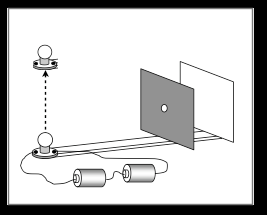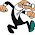Monday, May 16, 2011

Light and Shadow: The best questions comes from students

Last year, I watched a lot of video of students working through worksheets on topic of light and shadow. Their questions led me to think about this problem:Students are supposed to think about "where" the light goes when you raise it. But one group went about thinking about what would happen to the shape and size of the spot of light as you move it from aligned with the hole to farther and farther up or down? Students questions are the best ones.

So, How does the size/shape of the bulb change as you move the bulb up and down? First give your intuitive guess... and then do some work to figure it out and convince someone else.

(I have three very different approaches to answering this. My most elegant came just last night as I was falling asleep.)

1.How high does the light go? Does it 'clear' the mask or can we assume the mask is infinitely large?

2.You can assume an infinitely large mask... so basically the question is how does size of spot light change as you move up and down (to any location along the vertical line)?... and of course the solution is more the point than the answer ;)

3.Okay, I'd say the spot (on the screen) moves downwards as the light source is moved upwards. I'm thinking about the rays of light leaving the source and which rays can make it through the hole. So originally there is a bright spot on the screen (ignoring diffraction) opposite the hole when the light is behind the hole. Then as the light source is raised the bright spot drops down the screen and moves across the table towards the mask. Thoughts?

4.Yeah, and this is what students are supposed to figure out--that the light moves down as you raise the bulb (ignoring the table, pretending both the mask and screen are infinitely large).

But the students also thought that the spot of light would stretch out as you moves it down (making it elongated). It sounds like you are assuming that the spot of light stays the same size (while it's on the screen, not on the table). If that's the case, how would you convince someone else of this -- that it doesn't change size? Or perhaps that it does change size?

5.That the students think the spot would elongate is something on which I'd follow up. I would ask them to explain why, and anticipate two possible explanations:

* Diffraction: Their intuition about diffraction may be kicking in, because a smaller hole (at least they are getting this part right) would diffract the light to a greater degree. This is good, but like Kevin -- "(ignoring diffraction)" -- I think you'd have to move the light *very far* up before the amount of the diffraction becomes significant relative to the size of the hole as seen by the light. And by that time the intensity of the light would be negligible anyway.

* Geometry: As the lightbulb moves up and the angle at which it sees the hole changes, it would be able to see more of the mask subtending a smaller angle. Thus the students might think that “more of the mask” means more of the hole as well. This would show a lack of development in their spatial reasoning. Think of what you would see if you tipped the screen rather than the mask.

Oh, and the answer is that the light has a constant width but a narrower height as it moves down the screen, right? It's the projection of the hole onto the screen… in math terms it would be a dot product, or "hole*screen*cos θ" (where θ goes from zero → 90 as you raise the lightbulb, therefore cos θ goes from 1 → zero). The closest physics analogy from AP/honors physics might be W=F*d, where W is max when F and d are parallel. Likewise the size of the hole/spot would be max when the normal vector of the screen and the light rays coming from the hole are parallel.

How to convince somebody though... one of my favorite methods for "what does it do" problems is to figure out the behavior at the extremes, and then interpolate for the intermediate behavior. This method doesn't give you rates of change, but it works well because nature doesn't like discontinuities so things tend to change gradually, be it slowly at first then faster, or faster then less so. In this problem, if the light is directly in front of the hole, the image of the hole would be the same shape as the hole. This, nobody will argue against. Now imagine that the light is infinitely high above the hole, so far as to be basically above the hole (d_light_to_hole is negligible compared to h_light). Then how many light rays will enter the hole? Zero, because the light is edge-on to the hole (think magnetic flux problems). So the spot of light goes from normal to zero: it shrinks until it disappears.

Not sure how convincing that argument is, though. Probably best just to set up an experiment and do it!

6.Hey Micah. It's a fun problem to explore, both the physics and the students. I encourage you to explore some more. You've got a lot of cool ideas and arguments laid. It can help to start making sketches, because as you said, there is a lot of geometry to think through.

You wrote: "That the students think the spot would elongate is something on which I'd follow up."

I'd follow up, too. It makes sense to think this based on shining flashlights onto walls, they do kind of stretch out as you change the angle. You seem to think there would be stretching, too, right? As you can it would be small in direction, and stay the same in other. So you think , more like shrinking rather than elongating. Is that right?

You wrote: "If the light is directly in front of the hole, the image of the hole would be the same shape as the hole. Nobody will argue against" -

Can you articulate why the hole would stay the same. I'm thinking, for example, if it stays the same size as the hole, how does a movie project work if it stays the same size?

Your ideas of limiting cases is a really cool strategy. I agree that less light would be getting through, but does less light could mean dimmer? You seem to think smaller, and I'm interested as to why.